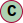# Class Matrix3

Serializable THREE.js Matrix3

#### Hierarchy-Diagram##### Legendclass

• Matrix3
• Matrix3

## Constructors

• Creates an identity matrix.

## Properties

elements: number[]

## Methods

• ### Deprecated

#### Returns number[]

• Sets the values of this matrix from the provided array or array-like.

#### Parameters

• ##### array: number[] | ArrayLike<number>

the source array or array-like.

• ##### `Optional` offset: number

(optional) offset into the array-like. Default is 0.

• ### Deprecated

• #### Returns Matrix3

• Inverts this matrix in place.

#### Returns Matrix3

• Sets this matrix as a 2D rotational transformation by theta radians. The resulting matrix will be:

``````cos(θ) -sin(θ) 0sin(θ) cos(θ)  00      0       1
``````

#### Parameters

• ##### theta: number

Rotation angle in radians. Positive values rotate counterclockwise.

#### Returns Matrix3

• Sets this matrix as a 2D scale transform:

``````x, 0, 0,0, y, 0,0, 0, 1
``````

#### Parameters

• ##### x: number

the amount to scale in the X axis.

• ##### y: number

the amount to scale in the Y axis.

#### Returns Matrix3

• Sets this matrix as a 2D translation transform:

``````1, 0, x,0, 1, y,0, 0, 1
``````

#### Parameters

• ##### x: number

the amount to translate in the X axis.

• ##### y: number

the amount to translate in the Y axis.

#### Returns Matrix3

• Multiplies this matrix by m.

#### Returns Matrix3

• Sets this matrix to a x b.

• ### Deprecated

This method has been removed completely.

#### Returns Matrix3

• Returns an array with the values of this matrix, or copies them into the provided array.

### Returns

The created or provided array.

#### Parameters

• ##### `Optional` array: number[]

(optional) array to store the matrix to. If this is not provided, a new array will be created.

• ##### `Optional` offset: number

(optional) optional offset into the array.

#### Returns Matrix3Tuple

• Copies he values of this matrix into the provided array-like.

### Returns

The provided array-like.

#### Parameters

• ##### `Optional` array: ArrayLike<number>

array-like to store the matrix to.

• ##### `Optional` offset: number

(optional) optional offset into the array-like.

#### Returns Matrix3

• Transposes this matrix in place.

#### Returns Matrix3

• Transposes this matrix into the supplied array r, and returns itself.

#### Returns Matrix3

• Create a matrix from array

Matrix3

Array